New and improved technical tools   Imaging lens selector    Visit

CNHomepage
/
Technical literature

Sort out

# Technical literature

• Categories:Resources
• Time of issue:2019-07-23 00:00:00
• Views:0
Description:
Description:
Information

Damage Threshold

1. Definition:

Colloquially speaking, Laser-Induced Damage Threshold (LIDT) represents the critical value of a laser intensity at which an optical device can withstand without being permanently damaged. The LIDT of an optical device depends largely on the type of laser used. A Continuous Wave (CW) Laser usually causes damage through thermal effects (absorption of the coating or substrate). Pulsed Lasers typically strip electrons from the lattice before causing thermal damage.

1. Criterias of LIDT:

The Damage Threshold can be expressed in the following ways: as for CW Lasers, the Average Power Density is usually used, its calculating method is as follow:

Average Power Density=Average Power (W)Area of Laser Spot where it's measured(cm2)=W/cm2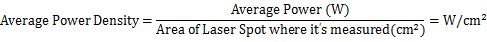As for Pulsed Lasers, the Energy Density and Peak Power Density is usually used, its calculating methods are as follow:

Energy Density=Energy of Monopulse (J)Area of Laser Spot where it's measured(cm2)=J/cm2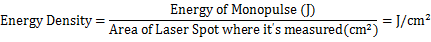Peak Power Density=Energy of Monopulse J/Pulse Width(s) Area of Laser Spot where it's measured(cm2)=W/cm2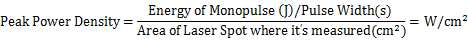1. Conditions of Use

How to choose a suitable method of evaluation for a certain condition?

A Continuous Wave (CW) Laser causes damage to an optical device mainly through thermal effect, its damage threshold is represented by the highest tolerable laser power density. To be on the safe side, for laser beams with a Gaussian intensity profile, its power density needed to be multiplied by two to accommodate the peak power density at the center of the beam.

The damage threshold of an optical device under a Pulsed Laser is usually expressed by the maximum tolerable pulse energy density. For Pulsed Laser with pulse width between microseconds and nanoseconds, the damage threshold is proportional to the square root of the pulse width. In the realm in between millisecond and CW, because it situate in the overlap range between Pulsed Laser and CW Laser, it is usually safer to compare both the average power with the CW threshold and the pulse energy density with the energy specification. In addition, for Pulsed Lasers, there may have some hot spots where the power is higher than other position, therefore it’s highly recommended to apply a safety factor of 2 or 3 to the power or power density before comparing to the LIDT. This factor, which represent the high power density at the center of beam, is also applicable for Gaussian shaped beams.

1. Conversion Equation

1. between different wavelengths

According to experience, the damage threshold measured under different wavelength will be scaled appropriately, and there is a linear relationship between them. When the wavelength decreases, the damage threshold will also decrease correspondingly. The empirical formula is:

Damage Threshold Required=Damage Threshold Measured X Wave Length RequiredWave Length Measured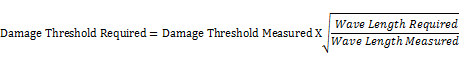For example, if the damage threshold measured at 1064nm is 1J/cm2, it can be inferred that the damage threshold measured at 532nm is 0.7J/cm2.

2. between different repetition frequencies

According to experience, when the repetition frequency of Pulsed Laser changes, the corresponding damage threshold will also change, the damage threshold decreases with the increase of repetition frequency. It can be assumed that when the repetition frequency increases by an order, the damage threshold decreases by half. Notice that we only need to consider the order of magnitude relationship of repetition frequency. For example, when the repetition frequency is 10Hz, the damage threshold measured is 1J/cm2, it can be inferred that the damage threshold under the condition of 10KHz repetition frequency is 1/23= 0.125J/cm2. (repetition frequency varies by 3 orders of magnitude).

3. between different pulse widths

According to experience, the longer the pulse width is, the more energy the optical device can bear. The relation formula is approximately as follow:

Damage Threshold Required=Damage Threshold Measured X  Pulse Width RequiredPulse Width Measured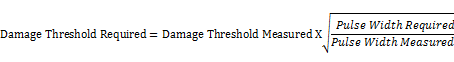For example, if the damage threshold measured under the condition of a laser beam with a pulse width of 20ns is 1J/cm2, it can be inferred that the damage threshold under the condition of a pulse width of 10ns is 0.7J /cm2.

1. Application Example

Test conditions: wavelength 1064nm, repetition frequency 10KHz, pulse width 12ns

Under the test laser condition, the damage threshold of the optical device is 11 J/cm2.

The conditions of application are: wavelength 532nm, repetition frequency 10Hz, pulse width 10ns

The first step is wavelength conversion, the damage threshold is 7.771J/cm2 at 532nm (the repetition frequency is 10 KHz and the pulse width is 12ns)

The second step is the conversion of repetition frequency. The difference between 10 KHz and 10 Hz is 3 orders of magnitude, so the damage threshold after conversion is 62.2J/cm2 (wavelength 532nm, repetition frequency 10Hz, pulse width 12ns)

The third step is pulse width conversion. From the measured pulse width of 12ns to the required pulse width of 10ns, according to the equation, the damage threshold is 56.8J/cm2 (wavelength 532nm, repetition frequency 10Hz, pulse width 10ns)

Summary of our ability: DHC has rich experience in lens coating, especially in the area of damage threshold, we have a complete detect method, can provide a reliable testing data. We can provide complete solutions according to different customer needs. Looking forward to your inquiry.